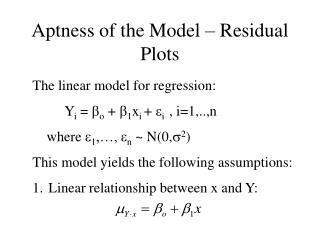# Aptness of the Model – Residual Plots - PowerPoint PPT PresentationDownload PresentationAptness of the Model – Residual Plots

Aptness of the Model – Residual Plots
Download Presentation## Aptness of the Model – Residual Plots

- - - - - - - - - - - - - - - - - - - - - - - - - - - E N D - - - - - - - - - - - - - - - - - - - - - - - - - - -
##### Presentation Transcript

1. Aptness of the Model – Residual Plots • The linear model for regression: • Yi = o + 1xi + i , i=1,..,n • where 1,…, n ~ N(0,2) • This model yields the following assumptions: • Linear relationship between x and Y:

2. 2. Equal variance for errors 3. Normally distributed errors 4. Independent errors The estimated error (residual) may be used to test whether these assumptions are satisfied (i.e., the model is appropriate)

3. Recall: Expectation and Variance of ei

4. This leads to the standardized residual If the assumptions are correct, the residuals should behave like normally distributed random variables and the standardized residuals like standard normal random variables.

5. To check the linearity and equal variance assumptions, plot eior ei* against xi or The use of standardized residuals ei* in these plots additionally provides some information about the normality assumption.

6. Good Residual Plots

7. Residual Plots w/ Nonlinear Data

8. Residual Plots w/ Unequal Varaiances

9. Residual Plots w/ Autocorrelation

10. To check the Independence assumption – In general, this is difficult to check. A plot of the residual vs. time of observation may be used. To check the Normality Assumption – A Normal Probability Plot (NPP) of the residuals may be used. Recall, a linear plot indicates that the normal distribution is consistent with the data (residuals).

11. Forming an NPP for the residuals: • Order the residuals: e(1),…,e(n) • Compute the normal percentiles: • Plot the (Pi, e(i)) pairs

12. What If Some of the Assumptions Are Violated? • Residual plot shows non-linearity – Fit a non-linear function (polynomial regression) or use a transformation to linearize (if possible) • Residual plot supports linearity, but shows a violation of the equal variances assumption – Use weighted least squares (WLS); give less weight to observation with larger variance. Consult the text Applied Linear Regression Models as referenced in Lecture 17.

13. The residuals support linearity and equal variances, but one of the standardized residuals is much greater (less) than +2 (-2) – This point is an outlier. If an assignable cause for this point may be found, throw it out and recalculate the regression parameters. If no assignable cause may be found, a MAD (minimum absolute deviation) approach may be used in place of L.S. (Least Squares). This approach, however, may be tedious.

14. A plot of the residuals vs. time show a violation of the independence assumption – A transformation may be used (if possible) or the time variable may be included in the model via multiple regression. See Applied Linear Regression Models. • A plot of the residuals vs. an independent variable not included in the model exhibits a definite pattern – Include this independent variable in a multiple regression analysis

15. Example: (12.4) Cont’d

16. Residuals vs. Predicted

17. Standardized Residual vs. Predicted

18. Scatter Plot

19. Revised Data Set – Outlier Omitted

20. Scatter Plot

21. Residuals vs. Predicted

22. Standardized Residuals vs. Predicted